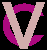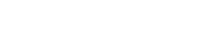Illustrated Dictionary of Computer Vision: GGabor filter
Gabor transform
Gabor wavelet
gaging
gait analysis
gait classification
gamma
gamma correction
gauge coordinates
Gaussian convolution
Gaussian curvature
Gaussian derivative
Gaussian distribution
Gaussian mixture model
Gaussian noise
Gaussian pyramid
Gaussian smoothing
Gaussian speckle
Gaussian sphere
gaze control
gaze direction estimation
gaze direction tracking
gaze location
Gelerkin approximation
generalized cone
generalized curve finding
generalized cylinder
generalized Hough transform
generalized order statistics filter
generate and test
generic viewpoint
genetic algorithm
genetic programming
genus
geodesic
geodesic active contour
geodesic active region
geodesic distance
geodesic transform
geographic information system
geometric compression
geometric constraint
geometric correction
geometric deformable model
geometric distance
geometric distortion
geometric feature
geometric feature learning
geometric feature proximity
geometric hashing
geometric invariant
geometric model
geometric model matching
geometric optics
geometric reasoning
geometric representation
geometric shape
geometric transformation
geon
gestalt
gesture analysis
gesture recognition
Gibbs sampling
GIF
GIS
glint
global
global positioning system
global structure extraction
global transform
golden template
grammar
grammatical representation
granulometric spectrum
granulometry
graph
graph cut
graph isomorphism
graph matching
graph model
graph partitioning
graph representation
graph searching
graph similarity
graph theoretic clustering
grassfire algorithm
grating
gray level X
gray scale
gray scale cooccurrence
gray scale correlation
gray scale distribution model
gray scale image
gray scale mathematical morphology
gray scale moment
gray scale morphology
gray scale similarity
gray scale texture moment
gray scale transformation
gray value X
greedy search
grey X
grid filter
ground following
ground plane
ground tracking
ground truth
grouping
grouping transform

Gabor filter: A filter formed by multiplying a complex oscillation by an elliptical Gaussian distribution (specified by two standard deviations and an orientation). This creates filters that are local, selective for orientation, have different scales and are tuned for intensity patterns (e.g. edges, bars and other patterns observed to trigger responses in the simple cells of the mammalian visual cortex) according to the frequency chosen for the complex oscillation. The filter can be applied in the frequency domain as well as the spatial domain .

Gabor transform: A transformation that allows a 1D or 2D signal (such as an image) to be represented as a weighted sum of Gabor functions.

Gabor wavelets: A type of wavelet formed by a sinusoidal function that is restricted by a Gaussian envelope function.

gaging: Measuring or testing. A standard requirement of industrial machine vision systems.

gait analysis: Analysis of the way in which human subjects move. Frequently used for biometric or medical purposes.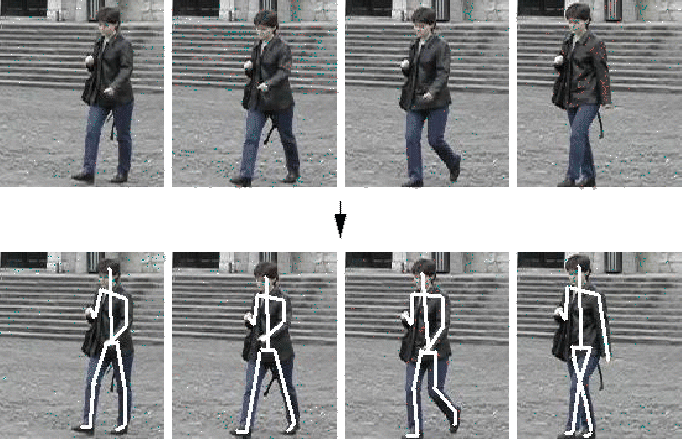gait classification: 1) Classification of different types of human motion (such as walking, running, etc.). 2) Biometric identification of people based on their gait parameters.

Galerkin approximation: A method for determining the coefficients of a power series solution for a differential equation.

gamma: Devices such as cameras and displays that convert between analogue (denoted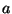) and digital (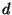) images generally have a nonlinear relationship betweenand. A common model for this nonlinearity is that the signals are related by a gamma curve of the form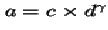, for some constant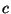. For CRT displays, common values of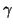are in the range 1.0-2.5.

gamma correction: The correction of brightness and color ratios so that an image has the correct dynamic range when displayed on a monitor.

gauge coordinates: A coordinate system local to the image surface itself. Gauge coordinates provide a convenient frame of reference for operators such as the gradient operator .

Gaussian convolution: See Gaussian smoothing .

Gaussian curvature: A measure of the surface curvature at a point. It is the product of the maximum and minimum of the normal curvatures in all directions through the point. See also mean curvature .

Gaussian derivative: The combination of Gaussian smoothing and a gradient filter . This results in a gradient filter that is less sensitive to noise.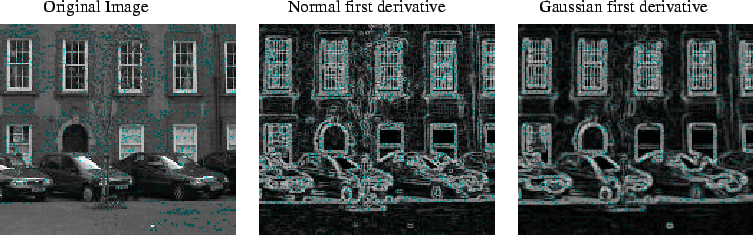Gaussian distribution: A probability density function with this distribution: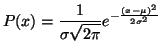whereis the mean and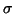is the standard deviation.

Gaussian mixture model: A representation for a distribution based on a combination of Gaussians. For instance, used to represent color histograms with multiple peaks. See expectation maximization.

Gaussian noise: Noise whose distribution is Gaussian in nature. Gaussian noise is specified by its standard deviation about a zero mean, and is often modeled as a form of additive noise .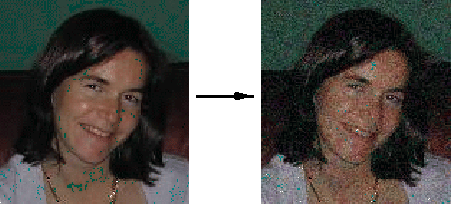Gaussian pyramid: A multi-resolution representation of an image formed by several images, each one a subsampled and Gaussian smoothed version of the original one at increasing standard deviation.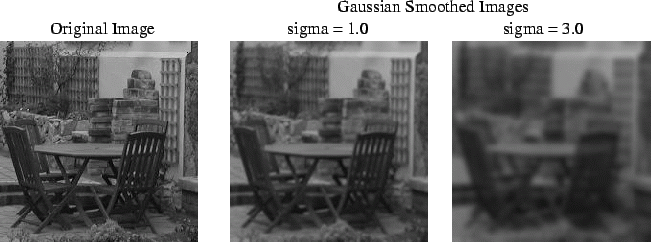Gaussian smoothing: An image processing operation aimed to attenuate image noise computed by convolution with a mask sampling a Gaussian distribution .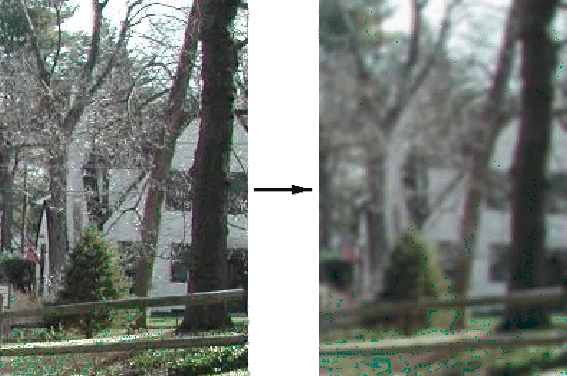Gaussian speckle: Speckle that has a Gaussian distribution .

Gaussian sphere: A sampled representation of a unit sphere where the surface of the sphere is defined by a number of triangular patches (often computed by dividing a dodecahedron). See also extended Gaussian image .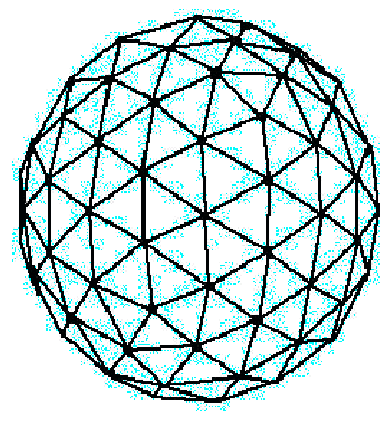gaze control: The ability of a human subject or a robot head to control their gaze direction.

gaze direction estimation: Estimation of the direction in which a human subject is looking. Used for human-computer interaction.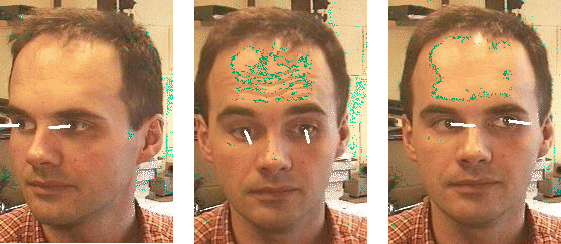gaze direction tracking: Continuous gaze direction estimation (e.g. in a video sequence or a live camera feed).

gaze location: See gaze direction estimation .

generalized cone: A generalized cylinder in which the swept curve changes along the axis.

generalized curve finding: A general term referring to methods that locate arbitrary curves. For example, see generalized Hough transform .

generalized cylinder: A volumetric representation where the volume is defined by sweeping a closed curve along an axis. The axis does not need to be straight and the closed curve may vary in shape as it is moved along the axis. For example a cylinder may be defined by moving a circle along a straight axis, and a cone may be defined by moving a circle of changing diameter along a straight axis.generalized Hough transform: A version of the Hough transform capable of detecting the presence of arbitrary shapes.

generalized order statistics filter: A filter in which the values within the filter mask are considered in increasing order and then combined in some fashion. The most common such filter is the median filter that selects the middle value.

generate and test: See hypothesize and verify .

generic viewpoint: A viewpoint such that small motions may cause small changes in the size or relative positions of features, but no features appear or disappear. This contrasts with a privileged viewpoint .

genetic algorithm: An optimization algorithm seeking solutions by refining iteratively a small set of candidates with a process mimicking genetic evolution. The suitability (fitness) of a set of possible solutions (population) is used to generate a new population until some conditions are satisfied (e.g. the best solution has not changed for a given number of iterations).

genetic programming: Application of genetic algorithms in some programming language to evolve programs that satisfy some evaluation criteria.

genus: In the study of topology , the number of "holes" in a surface. In computer vision, sometimes used as a discriminating feature for simple object recognition.

Gestalt: German for "shape". The Gestalt school of psychology, led by the German psychologists Wertheimer, Köhler and Koffka in the first half of the twentieth century, had a profound influence on perception theories, and subsequently on computer vision. Its basic tenet was that a perceptual pattern has properties as a whole, which cannot be explained in terms of its individual components. In other words, the whole is more than the sum of its parts. This concept was captured in some basic laws (proximity, similarity, closure, "common destiny" or good form, saliency), that would apply to all mental phenomena, not just perception. Much work on low-level computer vision, most notably on perceptual grouping and perceptual organization , has exploited these ideas. See also visual illusion .

geodesic: The shortest line between two points (on a mathematically defined surface).

geodesic active contour: An active contour model similar to the snake model in that it attempts to minimize an energy function between the model and the data, but which also incorporates a geometrical model.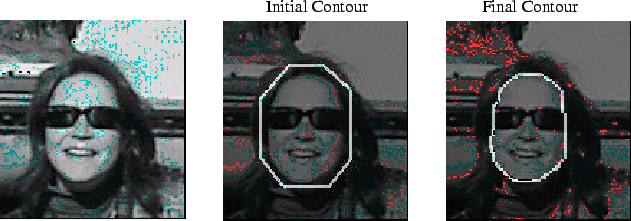geodesic active region: A technique for region based segmentation that builds on geodesic active contours by adding a force that takes into account information within regions. Typically a geodesic active region will be bounded by a single geodesic active contour.

geodesic distance: The length of the shortest path between two points along some surface. This is different from the Euclidean distance that takes no account of the surface. The following example shows the geodesic distance between Calgary and London (following the curvature of the Earth).geodesic transform: Assigns to each point the geodesic distance to some feature or class of feature.

geographic information system (GIS): A computer system that stores and manipulates geographically referenced data (such as images of portions of the Earth taken by satellite).

geometric compression: The compression of geometric structures such as polygons.

geometric constraint: A limitation on the possible physical arrangement/appearance of objects based on geometry. These types of constraints are used extensively in stereo vision (e.g. the epipolar constraint ), motion analysis (e.g. rigid motion constraint) and object recognition (e.g. focusing on specific classes of objects or relations between features).

geometric correction: In remote sensing , an algorithm or technique for correction of geometric distortion .

geometric deformable model: A deformable model in which the deformation of curves is based on the level set method and stops at object boundaries. A typical example is a geodesic active contour model.

geometric distance: In curve and surface fitting , the shortest distance from a given point to a given surface. In many fitting problems, the geometric distance is expensive to compute but yields more accurate solutions. Compare algebraic distance .

geometric distortion: Deviations from the idealized image formation model (for example, pinhole camera) of an imaging system. Examples include radial lens distortion in standard cameras.

geometric feature: A general term describing a shape characteristic of some data, that encompasses features such as edges , corners , geons , etc.

geometric feature learning: Learning geometric features from examples of the feature.

geometric feature proximity: A measure of the distance between geometric features, e.g. as by using the distance between data and overlaid model features in hypothesis verification .

geometric hashing: A technique for matching models in which some geometric invariant features are mapped into a hash table, and this hash table is used to perform the recognition.

geometric invariant: A quantity describing some geometric configuration that remains unchanged under certain transformations (e.g. cross-ratio, perspective projection ).

geometric model: A model that describes the geometric shape of some object or scene. A model can be 2D (e.g. polycurve ) or 3D (e.g. surface based models), etc.

geometric model matching: Comparison of two geometric models or of a model and a set of image data shapes, for the purposes of recognition.

geometric optics: A general term referring to the description of optics from a geometrical point of view. Includes concepts such as the simple pinhole camera model , magnification , lenses , etc.

geometric reasoning: Reasoning with geometric shapes in order to address such tasks as robot motion planning, shape similarity, spatial position estimation, etc.

geometric representation: See geometric model .

geometric shape: A shape that takes a relatively simple geometric form (such as a square, ellipse, cube, sphere, generalized cylinder , etc.) or that can be described as a combination of such geometric primitives.

geometric transformation: A class of image processing operations that transform the spatial relationships in an image. They are used for the correction of geometric distortions and general image manipulation. A geometric transformation requires the definition of a pixel coordinate transformation together with an interpolation scheme. For example, a rotation does:geon: GEometrical iON. A basic volumetric primitive proposed by Biederman and used in recognition by components . Some example geons are:gesture analysis: Basic analysis of video data representing human gestures preceding the task of gesture recognition .

gesture recognition: The recognition of human gestures generally for the purpose of human-computer interaction. See also hand sign recognition .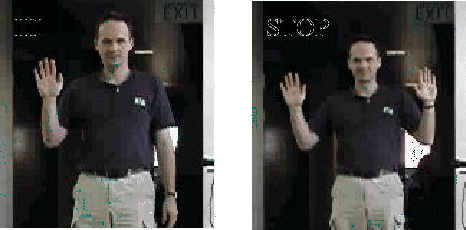Gibbs sampling: A method for probabilistic inference based on transition probabilities (between states).

GIF: Graphics Interchange Format. A common compressed image format based on the Lempel-Ziv-Welch algorithm.

GIS: See geographic information system .

glint: A specular reflection visible on a mirror -like surface.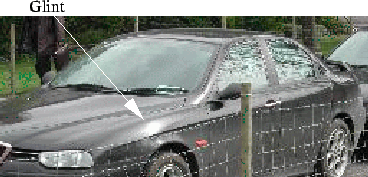global: A global property of a mathematical object is one that depends on all components of the object. For example, the average intensity of an image is a global property, as it depends on all the image pixels.

global positioning system (GPS): A system of satellites that allow the position of a GPS receiver to be determined in absolute Earth-referenced coordinates. Accuracy of standard civilian GPS is of the order of meters. Greater accuracy is obtainable using differential GPS.

global structure extraction: Identification of high level structures/relationships in an image (e.g. symmetry detection ).

global transform: A general term describing an operator that transforms an image into some other space. Sample global transforms include the discrete cosine transform , the Fourier transform , the Haar transform , the Hadamard transform , the Hartley transform , histograms , the Hough transform , the Karhunen-Loeve transform , the Radon transform , and the wavelet transform .

golden template: An image of an unflawed object/scene that is used within template matching to identify any deviations from the ideal object/scene.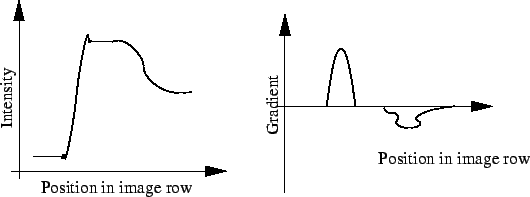gradient based flow estimation: Estimation of the optical flow based on gradient images. This computation can be done directly through the computation of a time derivative as long as the movement between frames is quite small. See also the aperture problem .

gradient descent: An iterative method for finding the (local) minimum of a function.

gradient filter: A filter that is convolved with an image to create an image in which every point represents the gradient in the original image in an orientation defined by the filter. Normally two orthogonal filters are used and by combining these a gradient vector can be determined for every point. Common filters include Roberts cross gradient operator , Prewitt gradient operator and the Sobel gradient operator . The Sobel horizontal gradient operator gives:gradient image: See edge image .

gradient magnitude thresholding: Thresholding of a gradient image in order to identify `strong' edge points .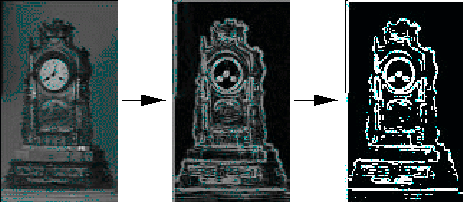gradient matching stereo: An approach to stereo matching in which the image gradients (or features derived from the image gradients) are matched.

gradient operator: An image processing operator that produces a gradient image from a gray scale input image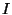. Depending on the usage of the term, the output could be 1) the vectors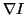of theand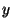derivatives at each point or 2) the magnitudes of these gradient vectors. The usual role of the gradient operator is to locate regions of strong gradients that signals the position of an edge . The figure below shows a gray scale image and its gradient magnitude image, where darker lines indicate stronger magnitudes. The gradient was calculated using the Sobel operator .gradient space: A representation of surface orientations in which each orientation is represented by a pair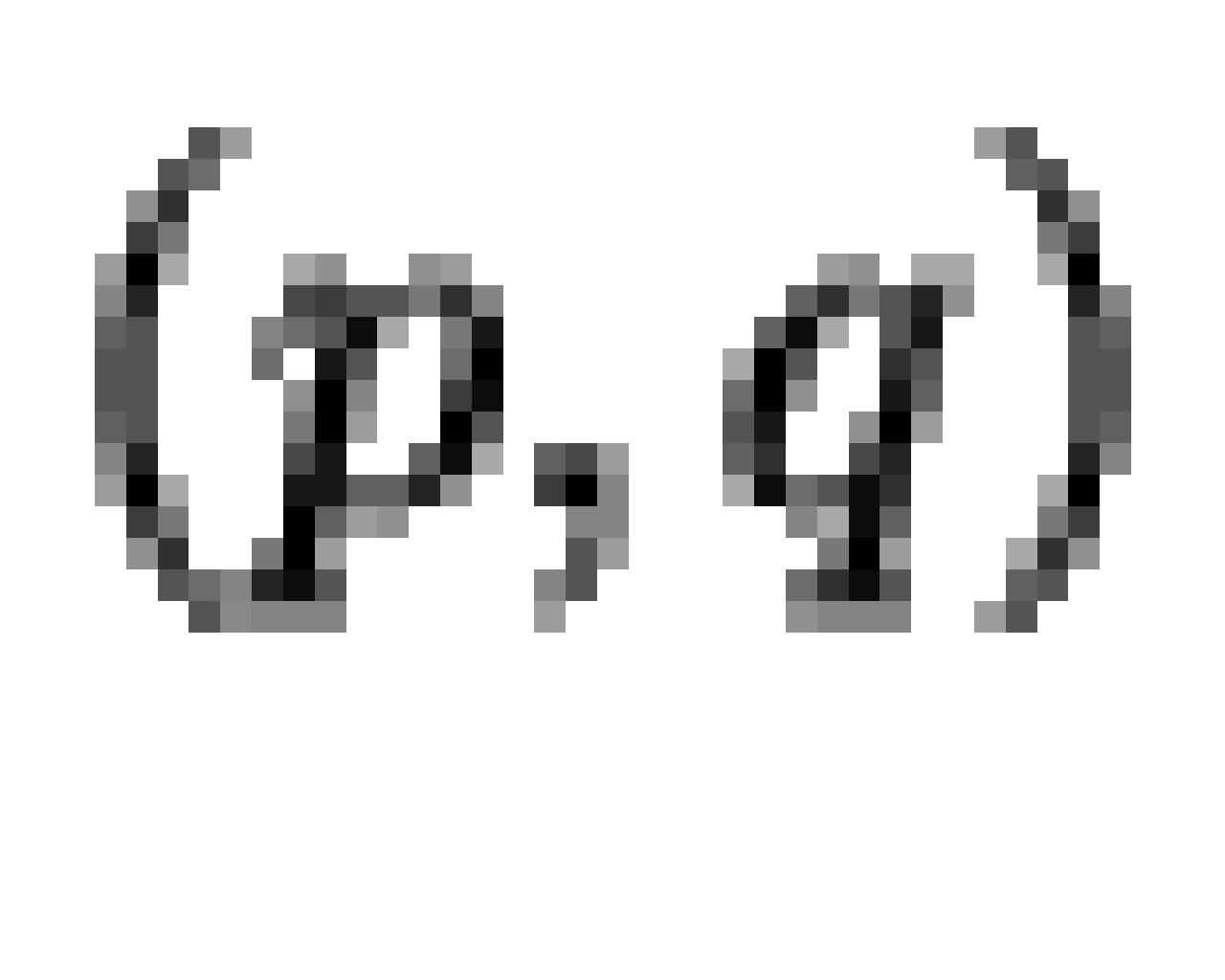where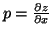and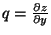(where theaxis is aligned with the optical axis of the viewing device).gradient vector: A vector describing the magnitude and direction of maximal change on an N-dimensional surface.

graduated non-convexity: An algorithm for finding a global minimum in a function that has many sharp local minima (a non-convex function). This is achieved by approximating the function by a convex function with just one minimum (near the global minimum of the non-convex function) and then gradually improving the approximation.

grammar: A system of rules constraining the way in which primitives (such as words) can be combined. Used in computer vision to represent objects where the primitives are simple shapes, textures or features.

grammatical representation: A representation that describes shapes using a number of primitives that can be combined using a particular set of rules (the grammar).

granulometric spectrum: The resultant distribution from a granulometry .

granulometry: The study of the size characteristics of a set (e.g. the size of a set of regions). Most normally this is achieved by applying a series of morphological openings (with structured elements of increasing size) and then studying the resultant size distributions.

graph: A graph is formed by a set of vertices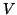and a set of edges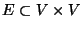linking pairs of vertices. Vertices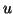and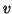are neighbors ifor. See graph isomorphism , subgraph isomorphism . This is a graph with five nodes: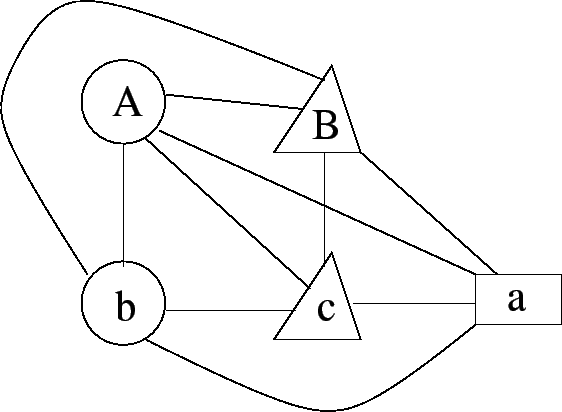graph cut: A partition of the vertices of a directed graphinto two disjoint setsand. The cost of the cut is the costs of all the edges that go from a vertex into a vertex in.

graph isomorphism: Two graphs are isomorphic if there exists a mapping (bijection) between their vertices that makes the edge sets identical. Determining whether two graphs are isomorphic is the graph isomorphism problem and is believed to be NP-complete . These small graphs are isomorphic with A:b, C:a, B:c: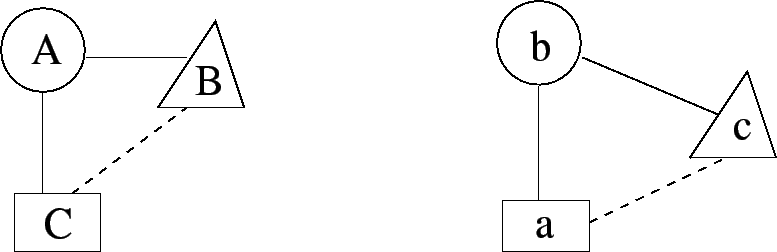graph matching: A general term describing techniques for comparing two graph models . These techniques may attempt to find graph isomorphisms , subgraph isomorphisms , or may just try to establish similarity between graphs.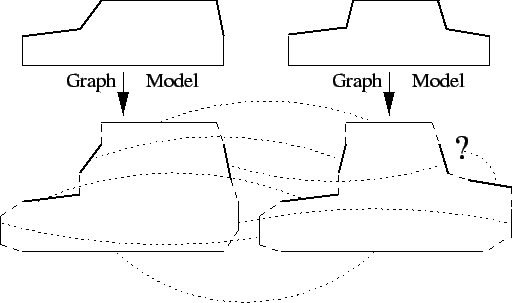graph model: A model of data in terms of a graph. Typical uses in computer vision include object representation (see graph matching ) and edge gradients (see graph searching ).

graph partitioning: The operation of splitting a graph into subgraphs satisfying some criteria. For example we might want to partition a graph of all polygonal edge segments in an image into subgraphs corresponding to objects in the scene.

graph representation: See graph model .

graph searching: Search for a specific node or path through a graph . Used, among other things, for border detection (e.g. in an edge gradient image) and object identification (e.g. decision trees).

graph similarity: The degree to which two graph representations are similar. Typically (in computer vision) these representations will not be exactly the same and hence a double subgraph isomorphism may need to be found to evaluate similarity.

graph theoretic clustering: Clustering algorithms that use concepts from graph theory, in particular leveraging efficient graph-theoretic algorithms such as maximum flow.

grassfire algorithm: A technique for finding a region skeleton based on wave propagation. A virtual fire is lit on all region boundaries and the skeleton is defined by the intersection of the wave fronts.grating: See diffraction grating .

gray level . . .: See gray scale . . .

gray scale: A monochromatic representation of the value of a pixel. Typically this represents image brightness and ranges from 0 (black) to 255 (white).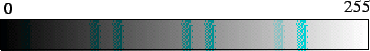gray scale co-occurrence: The occurrence of two particular gray levels some particular distance and orientation apart. Used in co-occurrence matrices .

gray scale correlation: The cross correlation of gray scale values in image windows or full images.

gray scale distribution model: A model of how gray scales are distributed in some image region . See also intensity histogram .

gray scale gradient: The rate of change of the gray levels in a gray scale image . See also edge , gradient image and first derivative filter .

gray scale image: A monochrome image in which pixels typically represents brightness values ranging from 0 to 255. See also gray scale .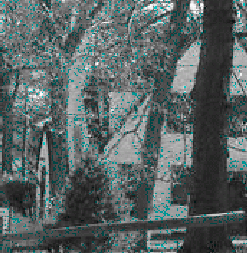gray scale mathematical morphology: The application of mathematical morphology to gray scale images . Each quantization level is treated as a distinct set where pixels are members of the set if they have a value greater than or equal to particular quantization levels.

gray scale moment: A moment that is based on image or region gray scales . See also binary moment.

gray scale morphology: See gray scale mathematical morphology.

gray scale similarity: See gray scale correlation .

gray scale texture moment: A moment that describes texture in a gray scale image (e.g. the Haralick texture operator describes image homogeneity).

gray scale transformation: A general term describing a class of image processing operations that apply to gray scale images , and simply manipulate the gray scale of pixels. Example operations include contrast stretching and histogram equalization .

gray value . . . : See gray scale . . .

greedy search: A search algorithm seeking to maximize a local criterion instead of a global one. Greedy algorithms sacrifice generality for speed. For instance, the stable configuration of a snake is typically found by an iterative energy minimization . The snake configuration at each step of the optimization can be found globally, by searching the space of all allowed configurations of all pixels simultaneously (a large space) or locally (greedy algorithm), by searching the space of all allowed configurations of each pixel individually (a much smaller space).

grey . . .: See gray . . .

grid filter: An approach to noise reduction where a nonlinear function of features (pixels or averages of a number of pixels) from the local neighborhood are used. Grid filters require a training phase where noisy data and corresponding ideal data are presented.

ground following: See ground tracking .

ground plane: The horizontal plane that corresponds to the ground (the surface on which objects stand). This concept is only really useful when the ground is roughly flat. The ground plane is highlighted here: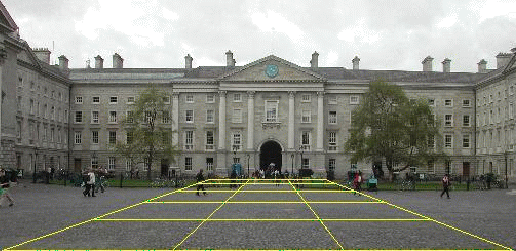ground tracking: A loosely defined term describing the robot navigation problem of sensing the ground plane and following some path.

ground truth: In performance analysis, the true value, or the most accurate value achievable, of the output of a specific instrument under analysis, for instance a vision system measuring the diameter of circular holes. Ground truth values may be known theoretically, e.g. from formulae, or obtained through an instrument more accurate than the one being evaluated.

grouping: 1) In human perception, the tendency to perceive certain patterns or clusters of stimuli as a coherent, distinct entity as opposed to a set of independent elements. 2) A whole class of segmentation algorithms is based on this idea. Much of this work was inspired by the Gestalt school of psychology. See also segmentation , image segmentation , supervised classification , and clustering.

grouping transform: An image analysis technique for grouping image features together (e.g. based on collinearity, etc.).